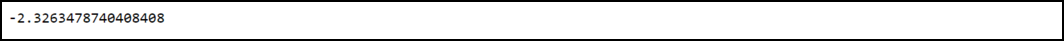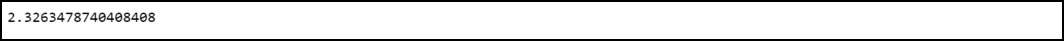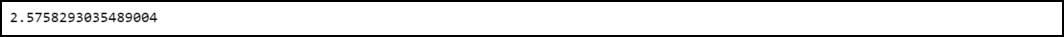Open in App
Not now

# How to Find the Z Critical Value in Python?

• Last Updated : 21 Feb, 2022

This article focuses on determining the Z-critical value in Python. When we conduct a hypothesis test, we get a test statistic as an outcome. In order to find out whether the result of the hypothesis test is statistically significant, we can compare the test statistic to a Z critical value. When the absolute value of the test statistic is larger than the Z critical value the outcome of the result is considered statistically significant.

Z critical value is a statistical term associated with the area under the standard normal model. It gives an idea about what will be the probability of any particular variable would have.

Syntax to install scipy library in python:

`pip3 install scipy`

Scipy is a python library used for scientific computation. It provides us scipy.stats.norm.ppf() function to compute the z-critical value.

scipy.stats.norm.ppf() function:

Syntax:

scipy.stats.norm.ppf(level)

Parameters:

level: It specifies the significance level to be used

## Z-value for the left-tailed test:

In this program, we are calculating the Z critical value for a left-tailed test with a significance level of .01.

Example:

## Python3

 `# Python program to find the z-value  ` `# in a left-tailed test ` ` `  `# Importing the library ` `import` `scipy.stats ` ` `  `# Determine the z-critical value ` `scipy.stats.norm.ppf(.``01``) `

Output:Z-value for the left-tailed test

The Z critical value comes out to be equal to -2.326. Therefore, if the test statistic is greater than this value, then the result of the test will be considered statistically significant.

## Z-value for the right-tailed test:

In this program, we are calculating the Z critical value for a right-tailed test with a significance level of .01.

Example:

## Python3

 `# Python program to find the ` `# z-value in a two-tailed test ` ` `  `# Importing the library ` `import` `scipy.stats ` ` `  `# Determine the z-critical value ` `scipy.stats.norm.ppf(``1``-``.``01``) `

Output:Z-value for the right-tailed test

The Z critical value comes out to be equal to 2.326. Therefore, if the test statistic is greater than this value, then the result of the test will be considered statistically significant.

## Z-value for the two-tailed test:

In this program, we are calculating the Z critical value for a two-tailed test with a significance level of .01.

Example:

## Python3

 `# Python program to find the z-value ` `# in a two-tailed test ` ` `  `# Importing the library ` `import` `scipy.stats ` ` `  `# Determine the z-critical value ` `scipy.stats.norm.ppf(``1``-``.``01``/``2``) `

Output:Z-value for the two-tailed test

Note that two critical values are there when we perform the two-tailed test. The Z critical value comes out to be equal to 2.575 which means two critical values are -2.575 and 2.575. Therefore, if the test statistic is lesser than -2.575 or greater than 2.575, then the result of the test will be considered statistically significant.

My Personal Notes arrow_drop_up
Related Articles